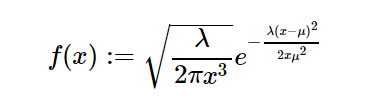sympy.stats.Wald() in Python

• Last Updated : 08 Jun, 2020

With the help of sympy.stats.Wald() method, we can get the continuous random variable which represents the inverse gaussian distribution as well as Wald distribution by using this method.Syntax : sympy.stats.Wald(name, mean, lamda)
Where, mean and lamda are positive number.

Return : Return the continuous random variable.

Example #1 :
In this example we can see that by using sympy.stats.Wald() method, we are able to get the continuous random variable representing inverse gaussian or wald distribution by using this method.

 # Import sympy and Waldfrom sympy.stats import Wald, densityfrom sympy import Symbol, pprint  z = Symbol("z")mean = Symbol("mean", positive = True)lamda = Symbol("lamda", positive = True)  # Using sympy.stats.Wald() methodX = Wald("x", mean, lamda)gfg = density(X)(z)  pprint(gfg)

Output :

2
-lamda*(-mean + z)
——————–
____ 2
___ _______ / 1 2*mean *z
\/ 2 *\/ lamda * / — *e
/ 3
\/ z
———————————————–
____
2*\/ pi

Example #2 :

 # Import sympy and Waldfrom sympy.stats import Wald, densityfrom sympy import Symbol, pprint  z = 0.86mean = 6lamda = 2.35  # Using sympy.stats.Wald() methodX = Wald("x", mean, lamda)gfg = density(X)(z)  pprint(gfg)

Output :

0.498668646362573
—————–
____
\/ pi

My Personal Notes arrow_drop_up